Win up to 100% scholarship on Aakash BYJU'S JEE/NEET courses with ABNAT Win up to 100% scholarship on Aakash BYJU'S JEE/NEET courses with ABNAT

# JEE Main 2022 June 26 – Shift 2 Chemistry Question Paper with Solutions

The JEE Main 2022 June 26th Shift 2 Chemistry question paper with solutions is given on this page to help students analyse their performance in the exam. They can verify their answers with the solutions written by our subject experts for JEE main chemistry question papers. This will make it easier for them to predict their scores in the examination. Get solutions for all the JEE main 2022 question papers on our website. They will help students improve their problem-solving skills, and further prepare for JEE main exams effectively.

## JEE Main 2022 26th June Evening Shift Chemistry Question Paper and Solutions

#### SECTION – A

Multiple Choice Questions: This section contains 20 multiple choice questions. Each question has 4 choices (1), (2), (3) and (4), out of which ONLY ONE is correct.

1. The number of radial and angular nodes in 4d orbital are, respectively

(A) 1 and 2

(B) 3 and 2

(C) 1 and 0

(D) 2 and 1

Sol. (i) In 4d n = 4 l = 2

Radial nodes = n – l – 1

= 4 – 2 – 1

= 1

Angular nodes = l

= 2

2. Match List I with List II

 List I Enzyme List II Conversion of A Invertase I Starch into maltose B Zymase II Maltose into glucose C Diastase III Glucose into ethanol D Maltase IV Cane sugar into glucose

Choose the most appropriate answer from the options given below.

(A) A-III, B-IV, C-II, D-I

(B) A-III, B-II, C-I, D-IV

(C) A-IV, B-III, C-I, D-II

(D) A-IV, B-II, C-III, D-I

Sol. (A) Invertase → Cane sugar into glucose

(B) Zymase → Glucose into ethanol

(C) Diastase → Starch into maltose

(D) Maltase → Maltose into glucose

3. Which of the following elements is considered as a metalloid?

(A) Sc

(B) Pb

(C) Bi

(D) Te

Sol. Tellurium is metalloid

4. The role of depressants in ‘Froth Floation method’ is to

(A) Selectively prevent one component of the ore from coming to the froth

(B) Reduce the consumption of oil for froth formation

(C) Stabilize the froth

(D) Enhance non-wettability of the mineral particles.

Sol. The role of depressants is to selectively prevent one component of the ore from coming to froth.

5. Boiling of hard water is helpful in removing the temporary hardness by converting calcium hydrogen carbonate and magnesium hydrogen carbonate to

(A) CaCO3 and Mg(OH)2

(B) CaCO3 and MgCO3

(C) Ca(OH)2 and MgCO3

(D) Ca(OH)2 and Mg(OH)2

Sol.

$$\begin{array}{l}Ca(HCO_3)_2\xrightarrow[]{\Delta}CaCO_3\downarrow+H_2O+CO_2\uparrow\end{array}$$
$$\begin{array}{l}Mg(HCO_3)_2\xrightarrow[]{\Delta}Mg(OH)_2+2CO_2\uparrow\end{array}$$

6. s-block element which cannot be qualitatively confirmed by the flame test is

(1) Li

(2) Na

(3) Rb

(4) Be

Sol. Beryllium does not give flame test because of its small size and high ionization energy the energy of flame is not sufficient to excite the electrons to higher energy level.

7. The oxide which contains an odd electron at the nitrogen atom is

(A) N2O

(B) NO2

(C) N2O3

(D) N2O5

Sol. The oxide of nitrogen which contains odd electron is NO2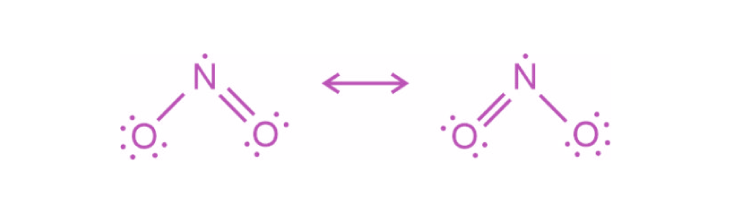8. Which one of the following is an example of disproportionation reaction?

$$\begin{array}{l}(A)\ 3MnO_{4}^{2-}+4H^+\to 2MnO_{4}^{-}+MnO_2+2H_2O\end{array}$$
$$\begin{array}{l}(B)\ MnO_{4}^{-}+4H^++4e^-\to MnO_2+2H_2O\end{array}$$
$$\begin{array}{l}(C)\ 10l^-+2MnO_{4}^{-}+16H^+\to 2Mn^{2+}+8H_2O+5l_2\end{array}$$
$$\begin{array}{l}(D)\ 8MnO_{4}^{-}+3S_2O_{3}^{2-}+H2O\to 8MnO_2+6SO_{4}^{2-}+2OH^-\end{array}$$

Sol.

$$\begin{array}{l}\overset{+6}{M}nO_{4}^{-2}\to \overset{+7}{M}nO_{4}^{-}\end{array}$$
$$\begin{array}{l}\overset{+6}{M}nO_{4}^{-2}\to \overset{+4}{M}nO_2\end{array}$$
$$\begin{array}{l}MnO_{4}^{-2}\end{array}$$
is an intermediate oxidation state and is converted into compounds having higher and lower oxidation states.

9. The most common oxidation state of Lanthanoid elements is +3. Which of the following is likely to deviate easily from +3 oxidation state?

(1) Ce(At. No. 58)

(2) La (At. No. 57)

(3) Lu (At. No. 71)

(4) Gd(At. No. 64)

Sol. Ce → [Xe]4f1 5d1 6s2

Ce+4 → [xe] 4f° 5d° 6s°

Cerium in +4 oxidation state acquires inert gas configuration.

10. The measured BOD values for four different water samples (A-D) are as follows:

A = 3 ppm; B = 18 ppm; C = 21 ppm; D = 4 ppm;. The water samples which can be called as highly polluted with organic wastes, are

(A) A and B

(B) A and D

(C) B and C

(D) B and D

Highly polluted water should have BOD value of 17 ppm or more.

11. The correct order of nucleophilicity is

(A) F > OH

$$\begin{array}{l}(B)\ H_2\ddot{\underset{..}O}>OH^-\end{array}$$
$$\begin{array}{l}(C)\ {R\ddot{\underset{..}{O}}H}>RO^-\end{array}$$
$$\begin{array}{l}(D)\ NH_{2}^{-}>NH_3\end{array}$$

Sol.

$$\begin{array}{l}\underset{Acid}{NH_3}\rightarrow\underset{Conjugate~ base}{NH_{2}^{-}}+H^+\end{array}$$

Conjugate base of acid is always a stronger nucleophile.

12. Oxidation of toluene to benzaldehyde can be easily carried out with which of the following reagents?

(A) CrO3/acetic acid, H3O+

(B) CrO3/acetic anhydride, H3O+

(C) KMnO4/HCl, H3O+

(D) CO/HCl, anhydrous AlCl3

Sol.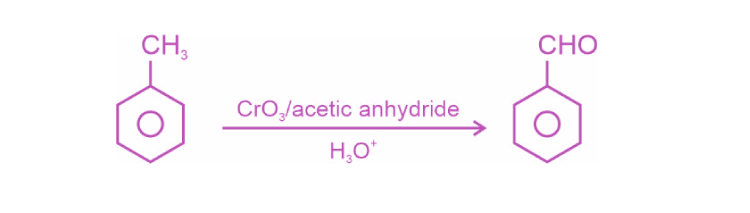13. The major product in the following reaction is: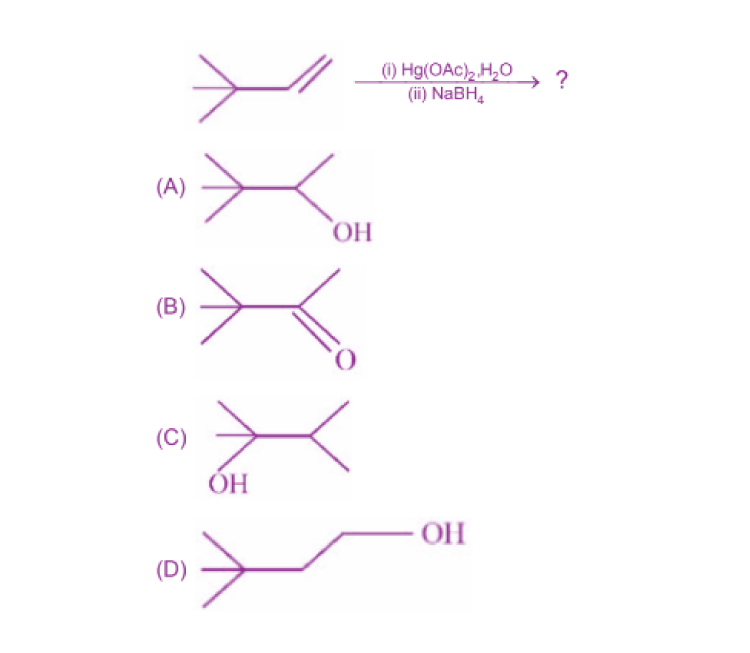Sol. Oxymercuration-demercuration follows Markovnikov’s addition of water without rearrangement.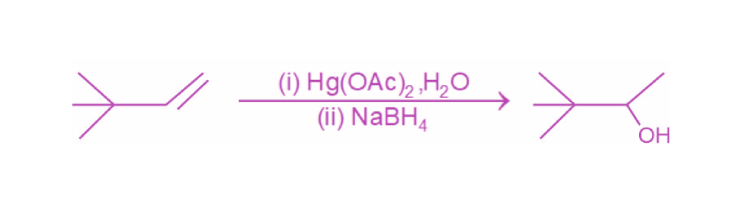14. Halogenation of which one of the following will yield m-substituted product with respect to methyl group as a major product?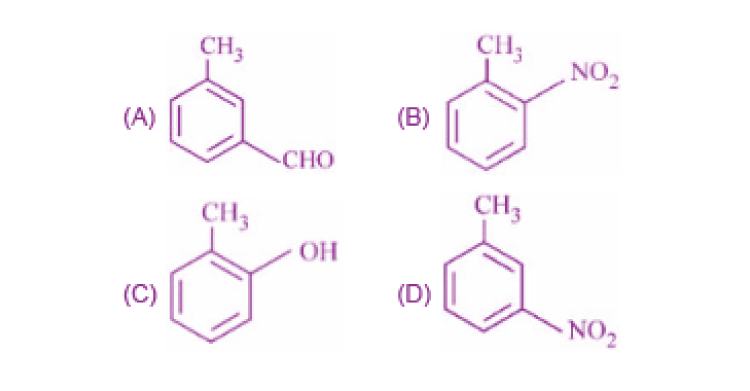Sol.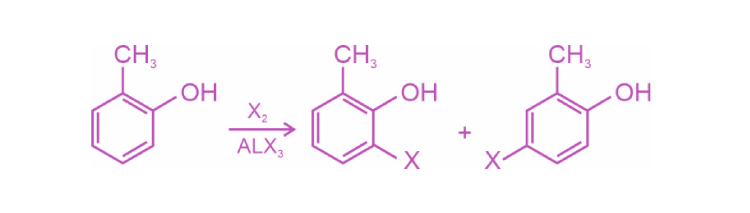Both products are meta with respect to –CH3.

15. The reagent, from the following, which converts benzoic acid to benzaldehyde in one step isSol. Benzoic acid can be converted to benzaldehyde in presence of MnO.

16. The final product ‘A’ in the following reaction sequence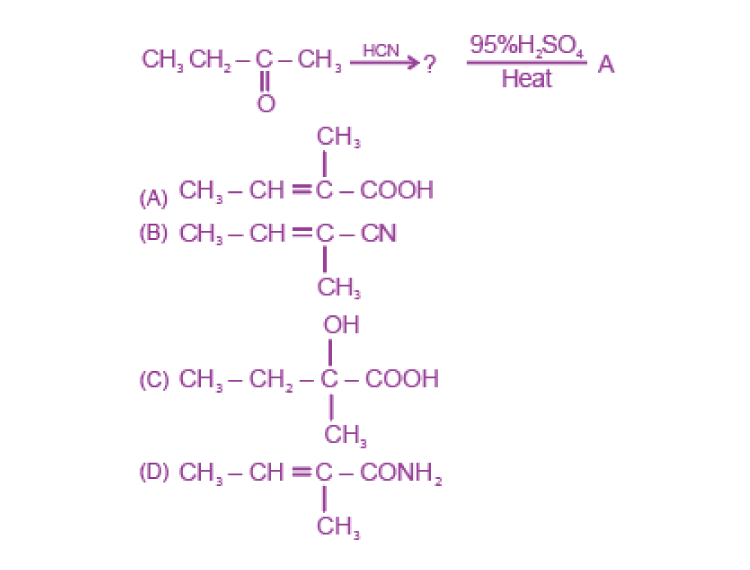Sol.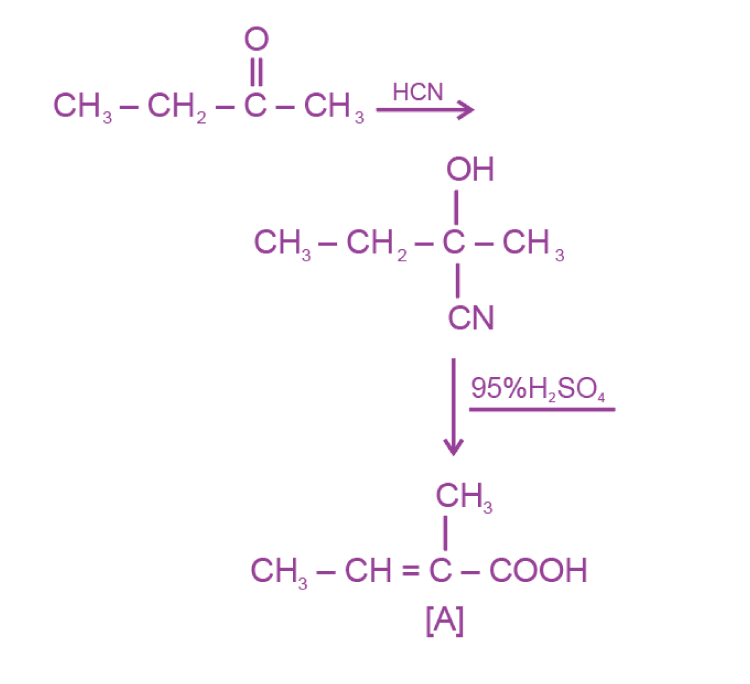17. Which statement is NOT correct for p-toluenesulphonyl chloride?

(A) It is known as Hinsberg’s reagent

(B) It is used to distinguish primary and secondary amines

(C) On treatment with secondary amine, it leads to a product, that is soluble in alkali

(D) It doesn’t react with tertiary amines

Sol.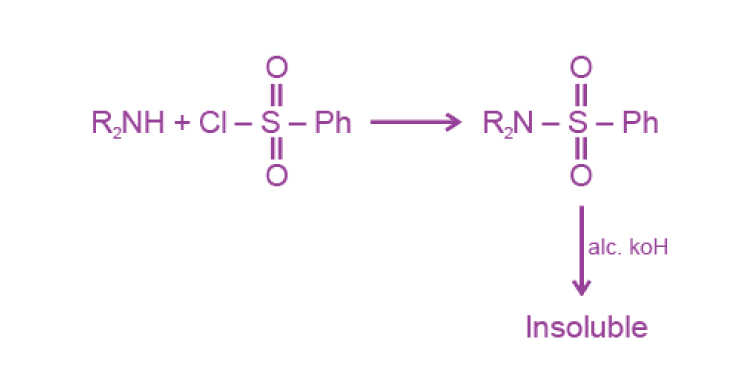18. The final product ‘C’ in the following series of reactions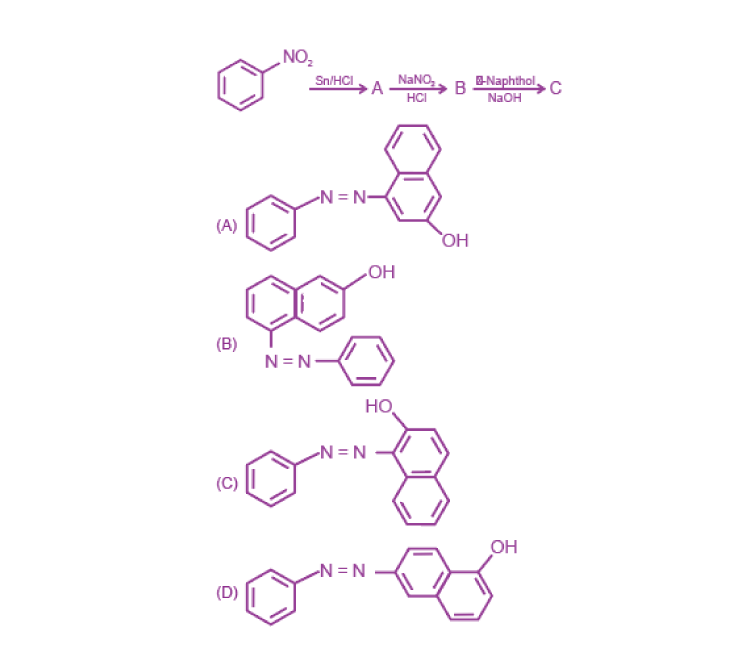Sol.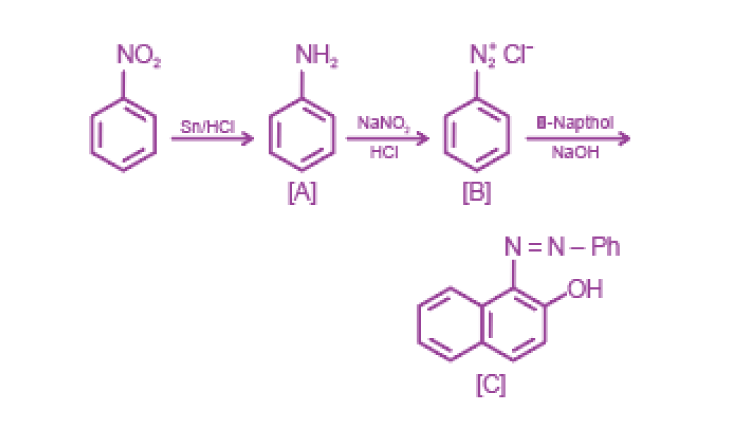19. Which of the following is NOT an example of synthetic detergent?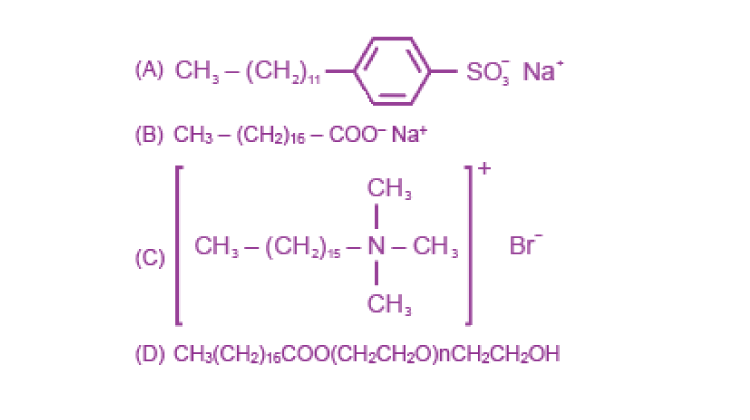Sol. CH3 – (CH2)16 – COO Na+

Sodium stearate is example of soap.

20. Which one of the following is a water soluble vitamin, that is not excreted easily?

(A) Vitamin B2

(B) Vitamin B1

(C) Vitamin B6

(D) Vitamin B12

Sol. Vitamin B12 is water soluble and not excreted easily.

#### SECTION – B

Numerical Value Type Questions: This section contains 10 questions. In Section B, attempt any five questions out of 10. The answer to each question is a NUMERICAL VALUE. For each question, enter the correct numerical value (in decimal notation, truncated/rounded-off to the second decimal place; e.g. 06.25, 07.00, –00.33, –00.30, 30.27, –27.30) using the mouse and the on-screen virtual numeric keypad in the place designated to enter the answer.

1. CNG is an important transportation fuel. When 100 g CNG is mixed with 208 g oxygen in vehicles, it leads to the formation of CO2 and H2O and produced large quantity of heat during this combustion, then the amount of carbon dioxide, produced in grams is _____. [nearest integer]

[Assume CNG to be methane]

Sol. CH4 + 2O2 → CO2 + 2H2O

$$\begin{array}{l}\begin{matrix}wt. of CH_4=100 ~\textup{g} \\wt. of O_2=208~\textup{g} \end{matrix}\end{array}$$
$$\begin{array}{l}n_{O_2}=\frac{208}{32}\end{array}$$
In this reaction O2 is limiting reagent

2 moles of O2 → 1 mole of CO2

1 mole of O2 → ½ mole of CO2

$$\begin{array}{l}\frac{208}{32}\ \text{mole of}\ O_2\rightarrow \frac{208}{32}\times\frac{1}{2}\ \text{mole of}\ CO_2\end{array}$$
$$\begin{array}{l}\rightarrow \frac{208}{32}\times\frac{1}{2}\times 44\ gm\ of\ CO_2\end{array}$$

→ 143 gm of CO2

2. In a solid AB, A atoms are in ccp arrangement and B atoms occupy all the octahedral sites. If two atoms from the opposite faces are removed, then the resultant stoichiometry of the compound is AxBy. The value of x is _____. [nearest integer]

Sol. A atoms are in CCP contribution of A is

A = 4

If atoms from opposite faces are removed then

$$\begin{array}{l}A=4-{x\times\frac{1}{x}}\end{array}$$

A = 3

Value of x = 3

3. Amongst SF4, XeF4, CF4 and H2O, the number of species with two lone pair of electrons is _____.

Sol.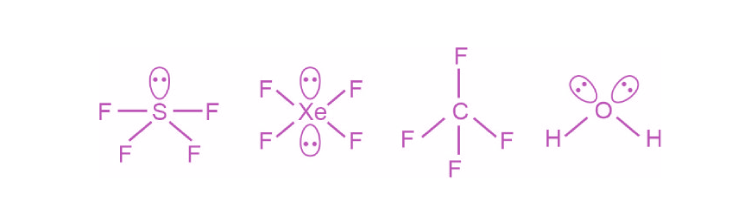XeF4 and H2O have 2 lone pairs.

4. A fish swimming in water body when taken out from the water body is covered with a film of water of weight 36 g. When it is subjected to cooking at 100 °C, then the internal energy for vaporization in kJ mol–1 is ________. [nearest integer]

[Assume steam to be an ideal gas. Given ΔvapHΘ for water at 373 K and 1 bar is 41.1 kJ mol–1; R = 8.31 J K–1 mol–1]

Sol.

$$\begin{array}{l}H_2\underset{36~\text{g}}{O}(l )\to H_2\underset{36g}{O}(g)\end{array}$$
(evaporation)

$$\begin{array}{l}n_{H_{2}O}=\frac{36}{18}=2\end{array}$$
Δng = 1 – 0 = 1

ΔUvap = ΔHvap – ΔngRT

= 41.1 – (1) × 8.31 × 10–3 × 373

= 41.1 – 3.099

= 38 kJ/mol

5. The osmotic pressure exerted by a solution prepared by dissolving 2.0 g of protein of molar mass 60 kg mol–1 in 200 mL of water at 27°C is _________ Pa. [Integer value]

(use R = 0.083 L bar mol–1 K–1)

Sol. π = i CRT (i = 1)

$$\begin{array}{l}\pi = \frac{2\times1000}{60\times10^3\times200}\times.083\times300\end{array}$$

π = 0.00415 atm

π = 415 Pa

6. 40% of HI undergoes decomposition to H2 and I2 at 300 K. ΔG° for this decomposition reaction at one atmosphere pressure is _____ J mol–1. [nearest integer]

(Use R = 8.31 J K–1 mol–1; log 2 = 0.3010, ln 10 = 2.3, log 3 = 0.477)

Sol.

$$\begin{array}{l}\begin{matrix}HI\rightleftharpoons &\frac{1}{2}H_2 &+\frac{1}{2}l_2 \\1 &0 &0 \\1-\alpha &\alpha/2 &\alpha/2 \\\end{matrix}\end{array}$$
$$\begin{array}{l}\Delta G^{\circ} =-RTInK\end{array}$$
$$\begin{array}{l}=-RTIn\frac{\left((\frac{\alpha }{2})^{1/2}\left(\frac{\alpha }{2}\right)^{1/2} \right )}{1-\alpha }\end{array}$$
$$\begin{array}{l}=RT In\frac{\alpha}{2(1-\alpha)}\end{array}$$
(α = 0.4)

$$\begin{array}{l}=-8.314\times300 In\frac{0.4}{2\times0.6}\end{array}$$
$$\begin{array}{l}=+8.314\times300~In3\end{array}$$
$$\begin{array}{l}=2735 ~\textup{J/mol}\end{array}$$

7.

$$\begin{array}{l}Cu(S)+Sn^{2+}(0.001M)\to Cu^{2+}(0.01M)+Sn(s)\end{array}$$

The Gibbs free energy change for the above reaction at 298 K is x × 10–1kJ mol–1. The value of x is ______.[nearest integer]

$$\begin{array}{l}[Given\ E_{cu^{2+}/Cu}^{\circleddash }=0.34V;E_{Sn^{2+}/Sn}^{\circleddash }=-0.14V;F=96500~\textup{C~mol}^{-1}]\end{array}$$

Sol.

$$\begin{array}{l}Cu+Sn^{2+}\to Cu^{2+}+Sn(s)\end{array}$$
$$\begin{array}{l}E^{\circ}_{cell}=E^{\circ}_{Ox}+E^{\circ}_{Red}\end{array}$$
$$\begin{array}{l}=-0.34-0.14\end{array}$$
$$\begin{array}{l}=-0.48~\textup{V}\end{array}$$
$$\begin{array}{l}E=E^{\circ}-\frac{0.0591}{2}log\frac{[Cu^{2+}]}{[Sn^{2+}]}\end{array}$$

= -0.48 – 0.0295 log 10

=-0.5095 V

$$\begin{array}{l}\Delta G=-nFE \end{array}$$
$$\begin{array}{l}=-2\times96500\times-0.5095~\textup{J/mol}\end{array}$$
$$\begin{array}{l}=98333.5\times10^{-3}~\textup{kJ/mol}\end{array}$$
$$\begin{array}{l}=983.3\times10^{-1} \textup{kJ/mol}\end{array}$$
$$\begin{array}{l}=983\times10^{-1} \textup{kJ/mol}\end{array}$$

8. Catalyst A reduces the activation energy for a reaction by 10 kJ mol–1 at 300 K. The ratio of rate constants,

$$\begin{array}{l}\frac{K’,Catalysed}{K,Uncatalysed}\end{array}$$
is ex. The value of x is ____.[nearest integer]

[Assume that the pre-exponential factor is same in both the cases Given R = 8.31 J K–1 mol–1]

Sol.

$$\begin{array}{l}In\frac{K’}{K}=\frac{E_a-E_{a}’}{RT}\end{array}$$
$$\begin{array}{l}=\frac{10\times10^3}{8.314\times300}\end{array}$$
$$\begin{array}{l}In\frac{K’}{K}=\frac{100}{8.314\times3}\end{array}$$
$$\begin{array}{l}\frac{K’}{K}=e^4\end{array}$$
$$\begin{array}{l}x=4\end{array}$$

9. Reaction of [Co(H2O)6]2+ with excess ammonia and in the presence of oxygen results into a diamagnetic product. Number of electrons present in t2g-orbitals of the product is _______.

Sol.

$$\begin{array}{l}[Co(H_2O)_6]^{+2}\xrightarrow[O_2]{NH_3}[Co(NH_3)_6]^{+3}+e^-\end{array}$$
$$\begin{array}{l}Co^{3+}\to 3d^6\end{array}$$

NH3 is a strong field ligand.

$$\begin{array}{l}3d^6\to t_{2g}^{6}eg^0\end{array}$$

10. The moles of methane required to produce 81 g of water after complete combustion is _____ × 10–2 mol. [nearest integer]

Sol.

$$\begin{array}{l}CH_4+2O_2\to CO_2+2H_2O\end{array}$$
$$\begin{array}{l}1\ \text{mol}\ CH_4\to\text{ 2 mole}\ H_2O\end{array}$$
$$\begin{array}{l}36\ \text{ gm}\ H_2O\to \text{1 mole}\ CH_4\end{array}$$
$$\begin{array}{l}81~gm~H_2O\to \frac{1}{36}\times81~mole~CH_4\end{array}$$
$$\begin{array}{l}\to 2.25 mole\end{array}$$
$$\begin{array}{l}\to 225\times 10^{-2}\end{array}$$

### Download PDF of JEE Main 2022 June 26 Shift 2 Chemistry Paper & Solutions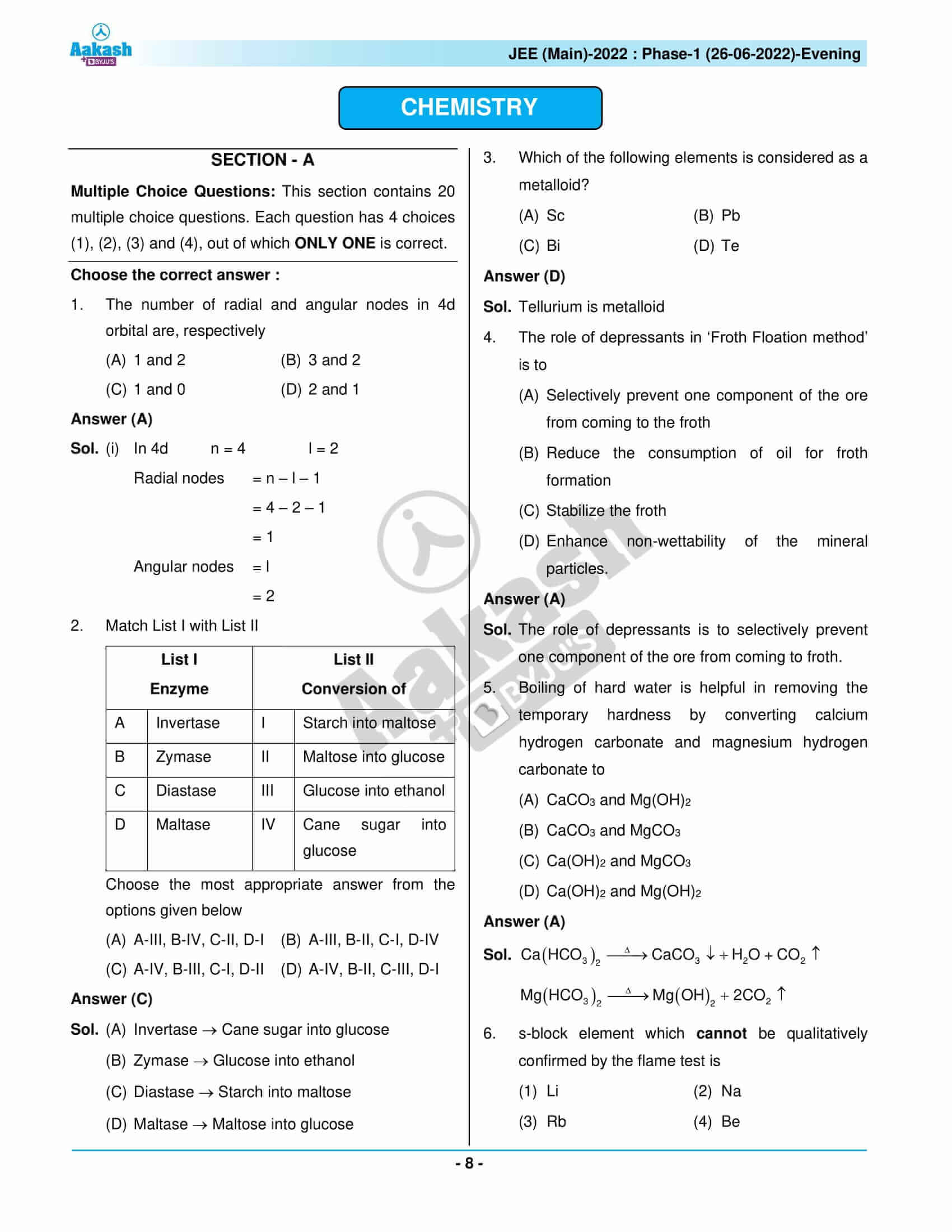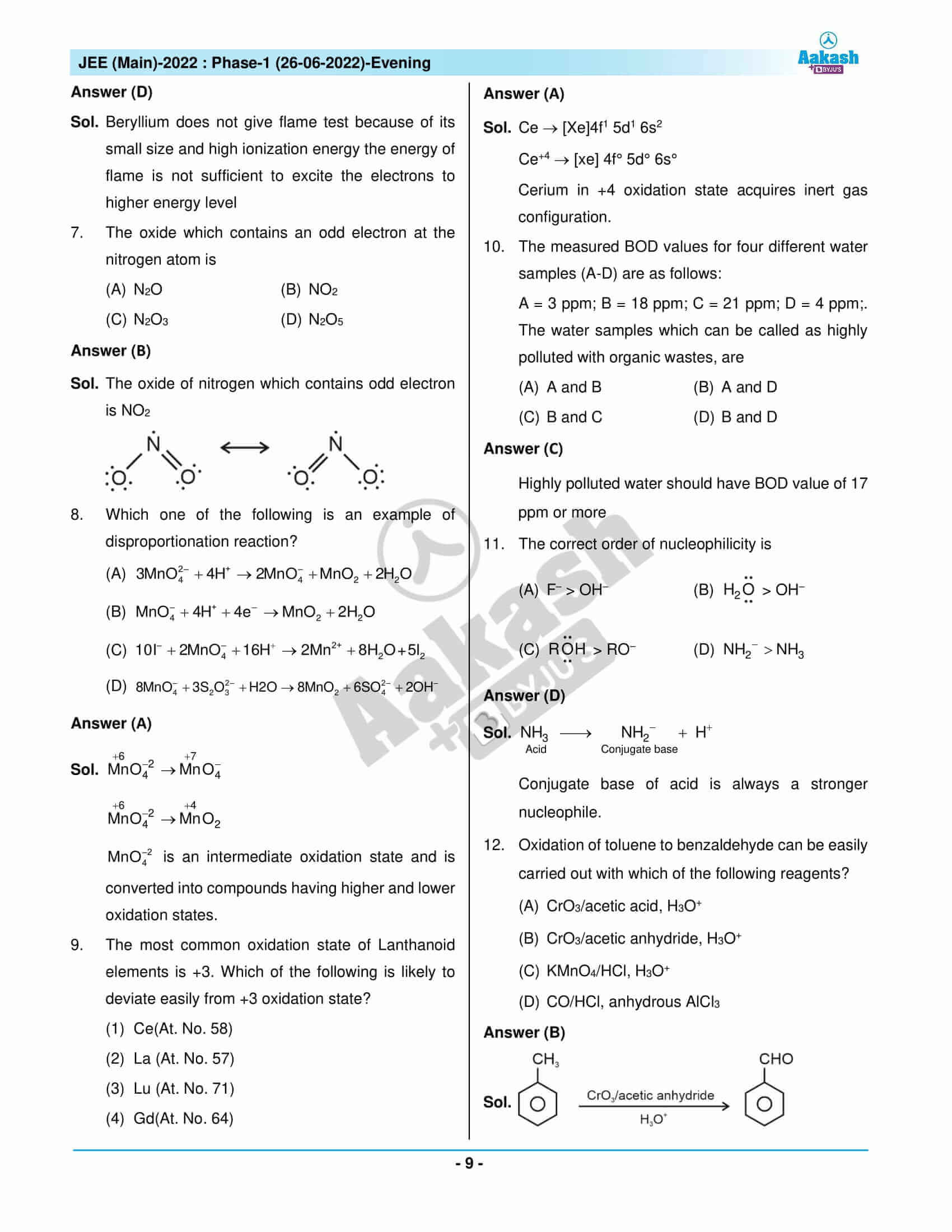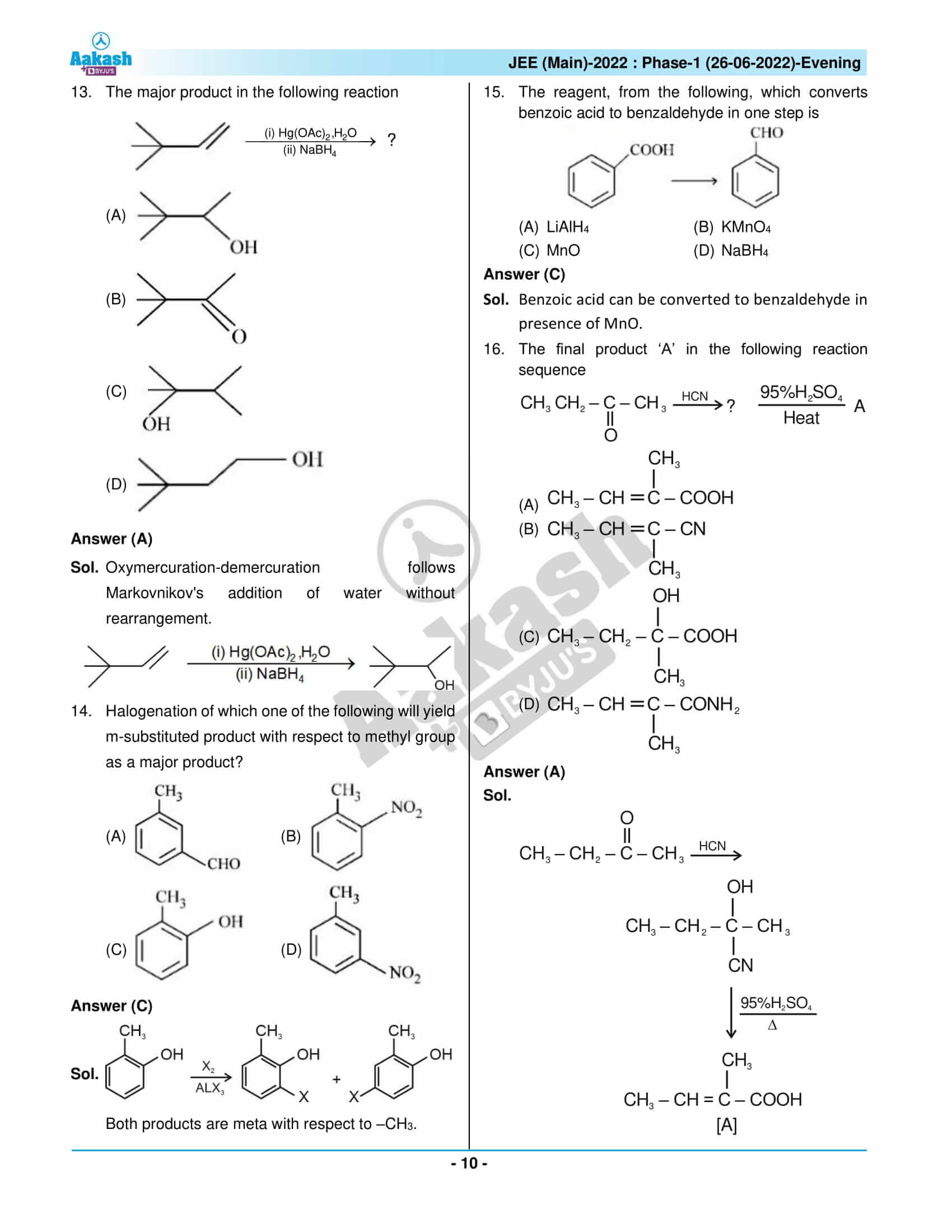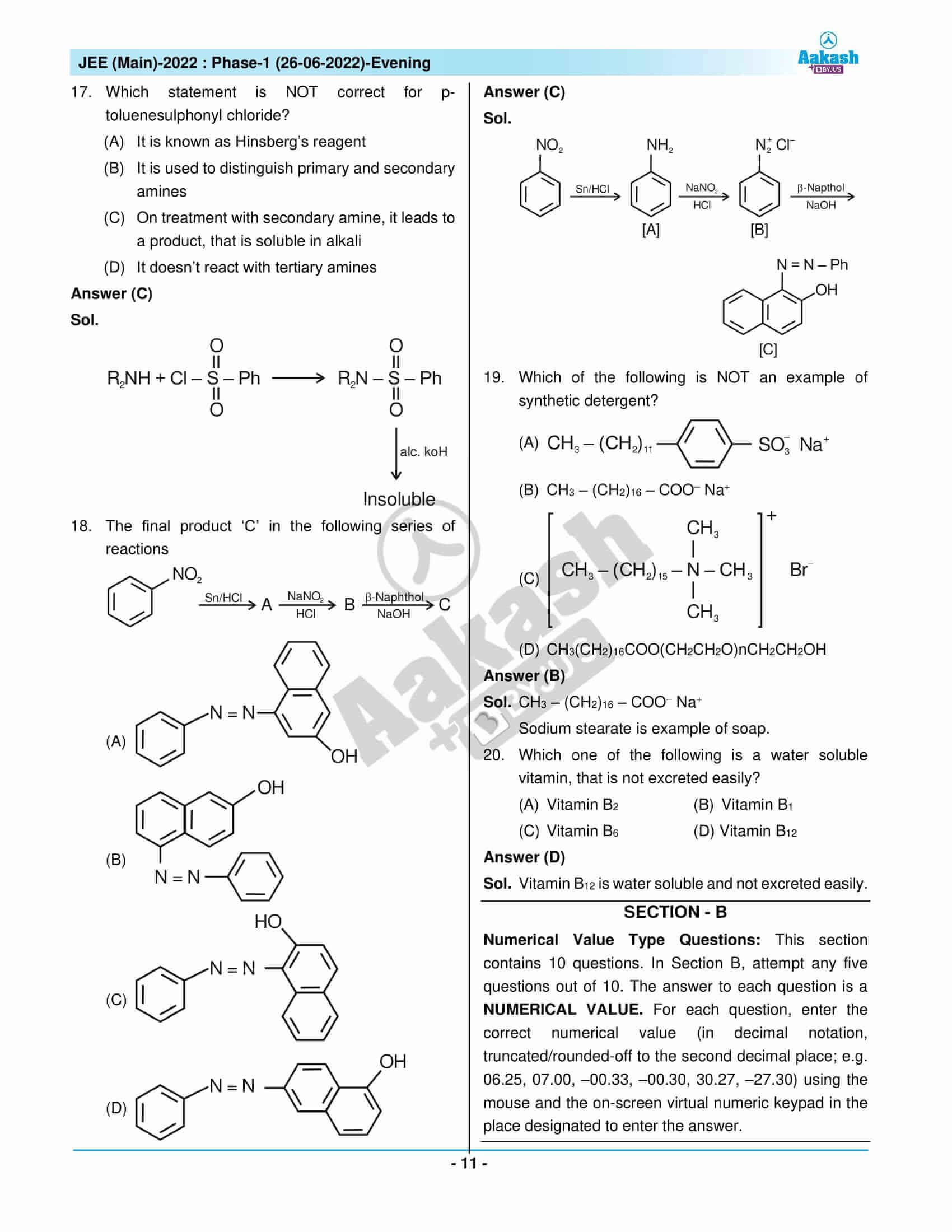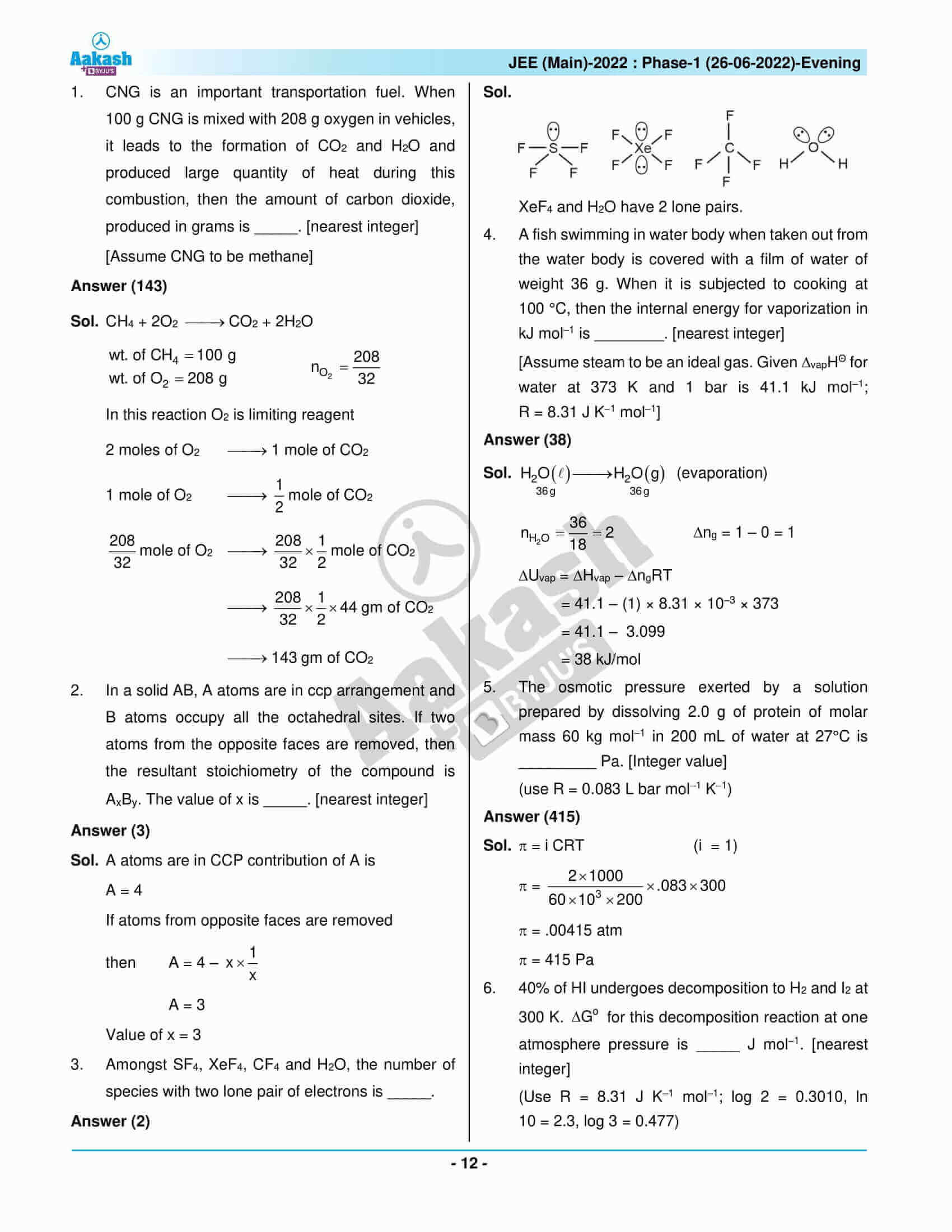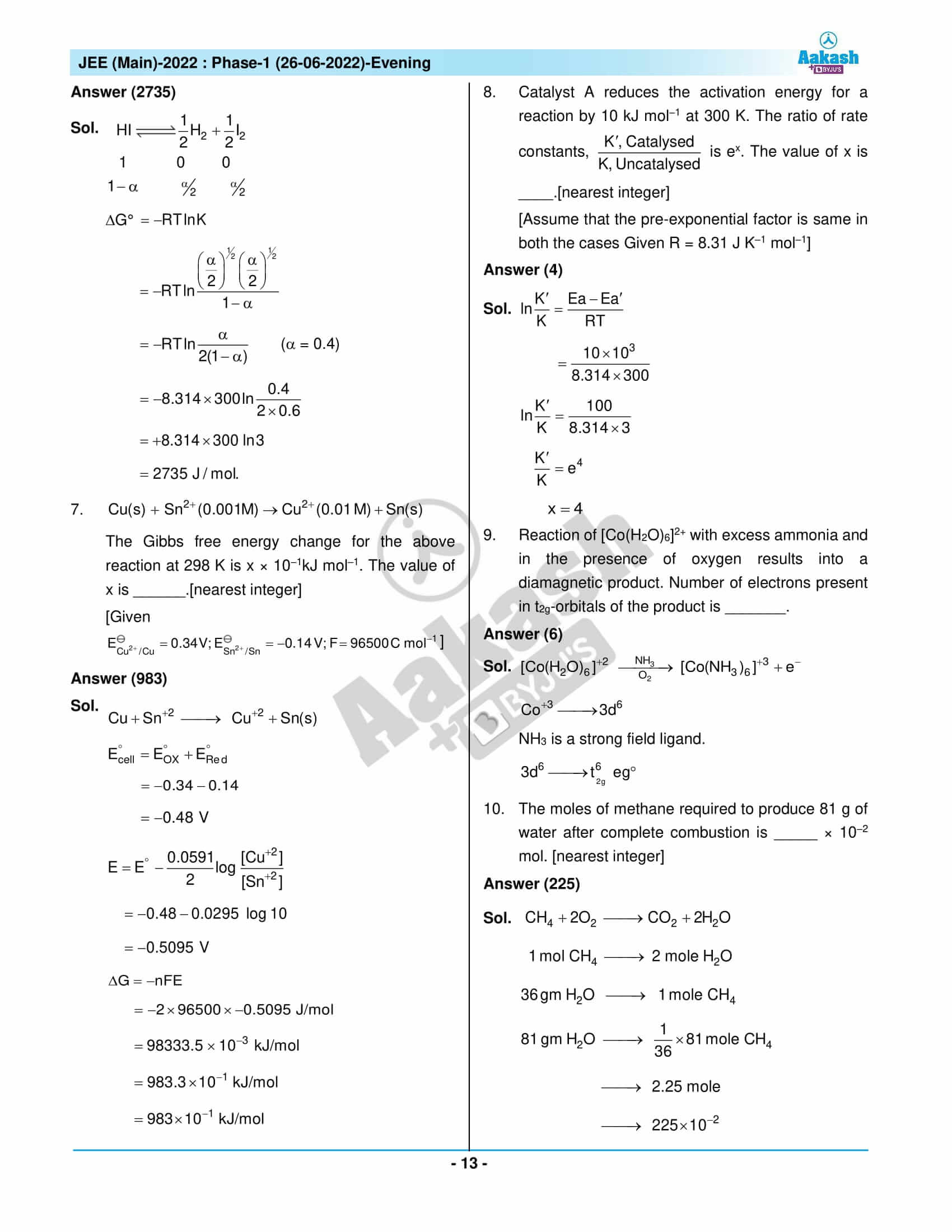## JEE Main Question Paper with Solutions – June 26th 2022 Shift 2

### JEE Main 2022 June 26 Shift 2 Question Paper – Physics Solutions### JEE Main 2022 June 26 Shift 2 Question Paper – Chemistry Solutions### JEE Main 2022 June 26 Shift 2 Question Paper – Maths Solutions## Frequently Asked Questions – FAQs

Q1

### What are the chapters included from Class 12 Chemistry in JEE Main 2022 June 26 shift 2 question paper?

The list of chapters from Class 12 in JEE Main 2022 June 26 shift 2 question paper are:
Biomolecules
Chemical Kinetics and Nuclear Chemistry
The P-Block Elements
The D and F-Block Elements
Aldehydes, Ketones and Carboxylic Acids
Electrochemistry
Surface Chemistry
The Solid State
Hydrocarbons
Amines
Chemistry In Everyday Life
Coordination Compounds
Solutions

Q2

### What chapters of Class 11 Chemistry are included in the JEE Main 2022 June 26th shift 2 question paper?

The list of chapters from Class 12 in the JEE Main 2022 June 26th shift 2 question paper are:
The S-Block Elements
Structure of Atom
Environmental Chemistry
Some Basic Concepts of Chemistry
Chemical Bonding and Molecular Structure
Thermodynamics

Q3

### What was the difficulty level of the Chemistry questions asked in JEE Main 2022 26th June second shift question paper?

Most of the Chemistry questions were either simple to moderate and one was difficult.

Q4

### If the questions are rated on a scale of 1 to 3, what was the difficulty level of Chemistry questions in JEE Main 2022 June 26 – Shift 2 Question Paper?

According to ratings done by the expert faculty, the difficulty level of Chemistry is 1.64 out of 3.

Q5

### How many difficult Chemistry questions were asked in JEE Main 2022 June 26 – shift 2 paper?

Of the 25 memory-based questions there was only 1 difficult question.